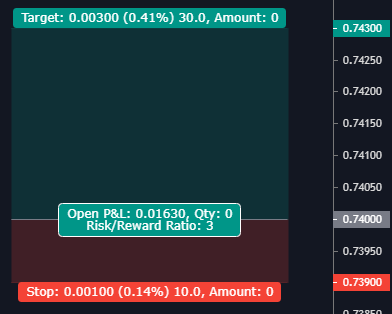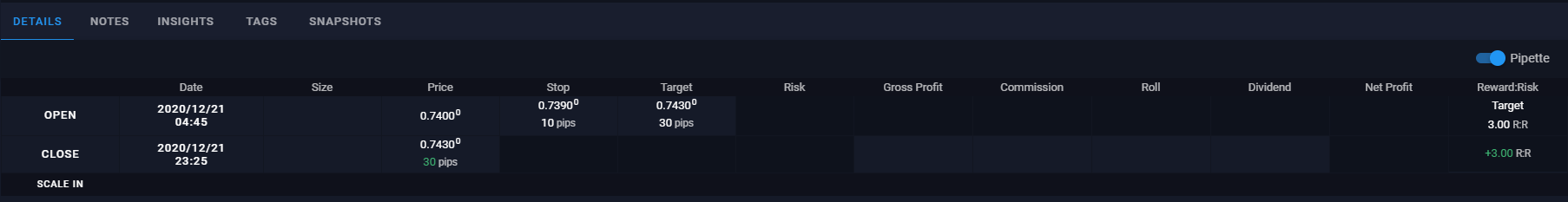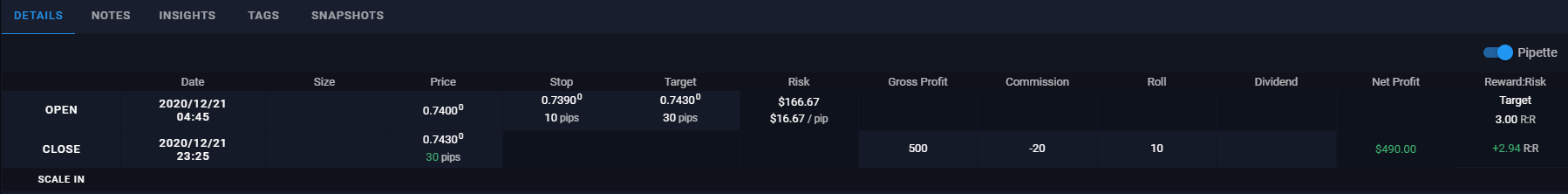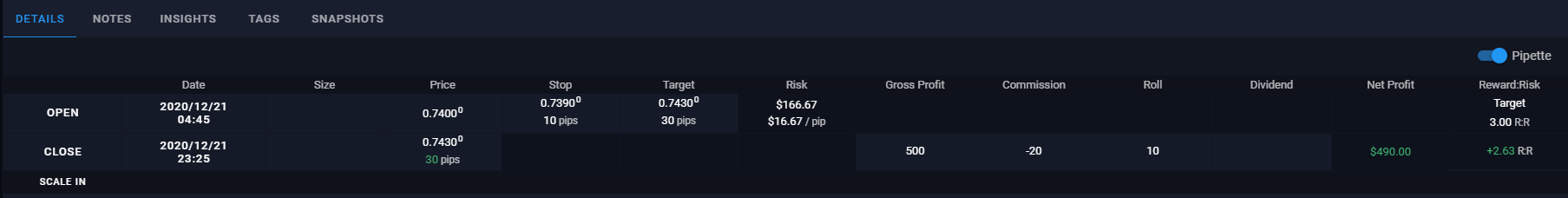and achieve long-term success as a professional trader.

# How is Reward:Risk Calculated

16 May, 21

The risk/reward ratio marks the prospective reward an investor can earn for every dollar they risk on an investment. Many investors use risk/reward ratios to compare the expected returns of an investment with the amount of risk they must undertake to earn these returns.

InvestopediaReward:Risk (R:R) Ratio is calculated by dividing your reward by your risk.

Trading Vault offers several methods to calculate your R:R Ratio based on how you wish to define your risk. You can choose which you prefer from within Trading Vault’s settings.

##### Method 1: Pip Reward:Risk

This method just looks at your pips and is irrelevant of your account and monetary risk, as it looks at the pure change in the price of an asset.

``Reward:Risk = Pip Reward / Pip Risk``

If you enter a target price, we can also calculate your Target R:R the same way. This is useful for comparing your Target against your Actual R:R.##### Method 2: Profit Reward:Risk

This method takes into consideration the actual money you have gained (loss) and risked. If you have not entered all the details to calculate this, then Trading Vault will revert to Pip R:R.

``````Reward:Risk = Net Profit / Financial Risk

where:

Net Profit = Gross + Commission + Roll + Dividends

Financial Risk = Pip Risked * Cost per pip

Cost per pip = Gross Profit / Pips Gained (Loss)``````##### Method 3: Profit Reward:Risk with commissions included in Risk

This method is the same as the Profit R:R but also includes the broker commissions in the amount risked.

``````Reward:Risk = Net Profit / Financial Risk

where:

Net Profit = Gross + Commission + Roll + Dividends

Financial Risk = (Pip Risked * Cost per pip) + Commissions

Cost per pip = Gross Profit / Pips Gained (Loss)``````